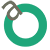###### Biography

I have completed Ph.D. in Numerical Analysis by 2016 from the Central University of Pondicherry, Pondicherry, India. I obtained a master degree in Mathematics from the University of Madras, Tamilnadu by 2012. I got a bachelor degree in Mathematics from the University of Madras by 2007.

I have taught quite a number of Engineering Mathematics courses over the years, and also engage research work till date. The outputs of my research works have been presented in quite a number of National and international conferences and as well been published in reputable journals. I am involved in the peer review of academic and professional Journals.

###### Research Interest

Numerical Analysis

###### Publications

1. Kalyanasundaram M, and D.K.R. Babajee “Comparing two techniques for developing higher order two-point iterative methods for solving quadratic equations” Algorthims, (Accepted)
2. Kalyanasundaram M, “Note on the Kung-Traub Conjecture for Traub-type two-point Iterative methods for Quadratic Equations” International Journal of Advance in Mathematics, Volume 2018, Number 2, Pages 112-118, 2018.
3. Kalyanasundaram M, “Sixth order Newton-type method for solving system of
nonlinear equations and its applications” Applied Mathematics E - Notes, 17(2017) 221-230. (Scopus)
4. Kalyanasundaram M. and J. Jayakumar. "Some efficient Newton-like solvers and
their application to Chandrasekhar’s equation and 1-D Bratu problem", Int. J. Appl.
Comput. Math. DOI:10.1007/s40819-016-0234-z, (2016), Springer
5. Kalyanasundaram M., D.K.R. Babajee and J. Jayakumar."An improvement to doublestep Newton method and its multi-step version for solving system of nonlinear equations and its applications", Numerical Algorithms, 74 (2) (2017) pp. 593-607 (Springer, SCI, IF = 1.37)
6. Kalyanasundaram M. and J. Jayakumar. "Higher Order Methods for Nonlinear Equations and their Basins of Attraction", Mathematics, 4(2) (2016),22 (Scopus, ESCI).
7. D.K.R. Babajee, Kalyanasundaram M., J. Jayakumar. On some improved Harmonic
Mean Newton-like methods for solving systems of nonlinear equations, Algorithms
8(2015), pp. 895-909. (Scopus, ESCI)
8. D.K.R. Babajee, Kalyanasundaram M., J. Jayakumar, A family of higher order multipoint iterative methods based on power mean for solving nonlinear equations, Afrika Matematika, 27(5) (2016) pp. 865-876. (Springer, Scopus)
9. Kalyanasundaram M., J. Jayakumar, Two new families of iterative methods for solving
nonlinear equations, Tamsui Oxf. J. Inf. Math. Sci., 31(1), (2017) 25-38 . (Scopus)
10. Kalyanasundaram M., J. Jayakumar, Class of modified Newton’s method for solving
nonlinear equations, Tamsui Oxf. J. Inf. Math. Sci., 30 (1) (2014) 91-100. (Scopus)
11. Kalyanasundaram M., J. Jayakumar, A fifth-order modified Newton-type method for
solving nonlinear equations, IJAER, 10(72) (2015) 83-86. (Scopus)
12. J. Jayakumar and Kalyanasundaram M. Power means based modification of Newton’s
method for solving nonlinear equations with cubic convergence, IJAMC,6(2)(2015) 1–6.
13. J. Jayakumar, Kalyanasundaram M., Generalized Power means modification of Newton’s method for Simple Roots of Nonlinear Equation, Int. J. P. Appl. Sci. Technol. 18(2)(2013) 45-51.
14. J. Jayakumar, Kalyanasundaram M., Modified Newton’s method using harmonic mean for solving nonlinear equation, IOSR journal of Mathematics 7(2013), 93–97.
Conference Papers
1. Kalyanasundaram M, “A modified Ostrowski’s method for solving nonlinear equation” International conference on Mathematical Computer Engineering, VIT University, Chennai, 3-4 Nov 2017.
2. Kalyanasundaram M., J. Jayakumar "Higher order Multi-point Iterative methods
and its Application to GPS and Nonlinear Differential Equations", Book of Abstracts
of the National Conference on Recent Developments in Mathematical Analysis and
its Applications, Pondicherry University, February 25-26, 2016.
3. Kalyanasundaram M., J. Jayakumar. A fifth-order modified Newton-type method for
solving nonlinear equations, Proceedings of the Interdisciplinary National Conference
on Soft computing and its Applications, Anna University, India, Vol. 1, 123-128,
2015.
4. J. Jayakumar, Kalyanasundaram M. Two Class of Sixth Order Modified Newton’s
Method for Solving Nonlinear Equations, Proceedings of the International Conference on Mathematics And its Applications, Anna University, India, 967-975, 2014.

## Submit your next article to SOAOJ Publishing!

#### and join the number of satisfied authors.x
Join as a Editor